# Parallel LINQ in C#

## Parallel LINQ (PLINQ) in C# with Examples:

In this article, I am going to discuss Parallel LINQ (PLINQ) in C# with Examples. Please read our previous article, where we discussed Interlocked vs. Lock in C# with Examples.

##### Parallel LINQ (PLINQ) in C#

If we have a collection, and if we want to use parallelism to process it, we have the option of using Parallel LINQ or PLINQ. Parallel LINQ (PLINQ) is basically the same as we have in LINQ. But with parallel functionality, we can define the maximum degree of parallelism and we can also use a cancellation token to cancel the operation and so on. To be able to process a sequence with PLINQ, we just need to use the AsParallel method.

##### Example to Understand Parallel LINQ in C#:

Let us understand Parallel LINQ in C# with an example. In the below example, we are creating a collection of integer numbers from 1 to 20 using Enumerable.Range method. Then using the LINQ Where Extension method, we are filtering the list of even numbers from the numbers collection. In the below example, we are not using Parallel LINQ, we are simply using LINQ.

```using System;
using System.Linq;

namespace ParallelLINQDemo
{
class Program
{
static void Main(string[] args)
{
//Creating a Collection of integer numbers
var numbers = Enumerable.Range(1, 20);

//Fetching the List of Even Numbers using LINQ
var evenNumbers = numbers.Where(x => x % 2 == 0).ToList();

Console.WriteLine("Even Numbers Between 1 and 20");
foreach (var number in evenNumbers)
{
Console.WriteLine(number);
}

}
}
}
```
###### Output: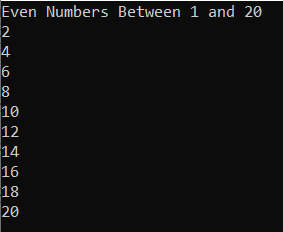Once you run the code, you will get the above output. Here, the following is the piece of code that filters the even numbers using LINQ.

var evenNumbers = numbers.Where(x => x % 2 == 0).ToList();

Now, let us see how to use Parallel LINQ in C# with the same example. As discussed earlier we just need to use the AsParallel method in order to process the sequence in parallel. For a better understanding, please have a look at the below code which shows both LINQ and PLINQ syntaxes to get the even numbers from the numbers collection. As you can see, with Parallel LINQ, after the collection or sequence, and before the Where extension method, we are calling the AsParallel Extension method.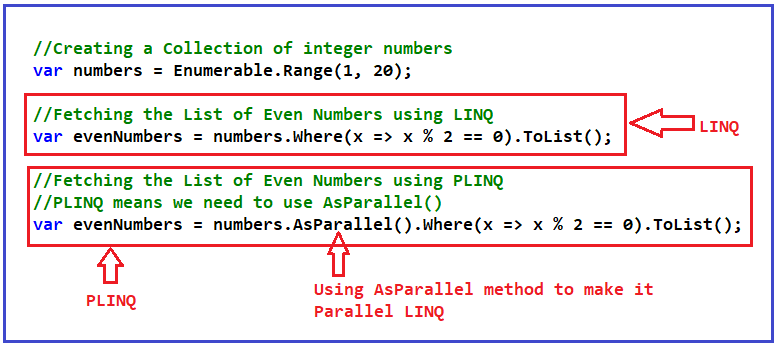So, this is as simple as it is. The following code uses parallelism. Now, the evaluations (i.e. x => x % 2 == 0) are going to be done in parallel i.e. the Where Extension method is going to be executed parallelly.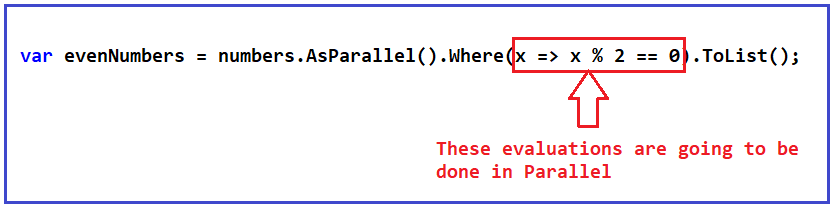Now, let us iterate over the evenNumbers collection and see the output. The following is the complete code example. The following example code is self-explained, so please go through the comment lines for a better understanding.

```using System;
using System.Linq;

namespace ParallelLINQDemo
{
class Program
{
static void Main(string[] args)
{
//Creating a Collection of integer numbers
var numbers = Enumerable.Range(1, 20);

//Fetching the List of Even Numbers using LINQ
//var evenNumbers = numbers.Where(x => x % 2 == 0).ToList();

//Fetching the List of Even Numbers using PLINQ
//PLINQ means we need to use AsParallel()
var evenNumbers = numbers.AsParallel().Where(x => x % 2 == 0).ToList();

Console.WriteLine("Even Numbers Between 1 and 20");
foreach (var number in evenNumbers)
{
Console.WriteLine(number);
}

}
}
}
```
###### Output: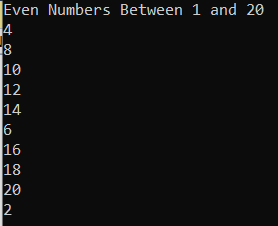You can observe the order of the numbers. They are in random order. This is because, we have already seen in the past that when we use parallelism, we typically cannot control the order of the operations i.e. we cannot simply predict the order of the output. Now, if you run the code multiple times, each time you might get a different order of the numbers.

##### How to Maintain the Original Order in Parallel LINQ?

If you want the output to be in order, then you need to use the AsOrdered() method after the AsParallel() method which means that after doing the operations in parallel, it will maintain the original order of the elements as they appeared in the sequence. For a better understanding, please have a look at the following image which shows how to use the AsOrdered method.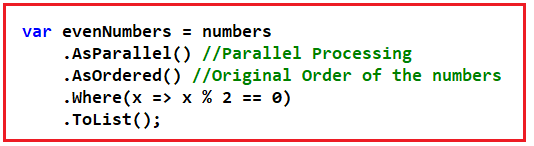The order will be the original order in which the elements are stored in the numbers collections. The following is the complete code. The following example code is self-explained, so please go through the comment lines for a better understanding.

```using System;
using System.Collections.Generic;
using System.Linq;

namespace ParallelLINQDemo
{
class Program
{
static void Main(string[] args)
{
//Creating a Collection of integer numbers
var numbers = Enumerable.Range(1, 20);

//Fetching the List of Even Numbers using PLINQ
//PLINQ means we need to use AsParallel()
var evenNumbers = numbers
.AsParallel() //Parallel Processing
.AsOrdered() //Original Order of the numbers
.Where(x => x % 2 == 0)
.ToList();

Console.WriteLine("Even Numbers Between 1 and 20");
foreach (var number in evenNumbers)
{
Console.WriteLine(number);
}

}
}
}
```
###### Output: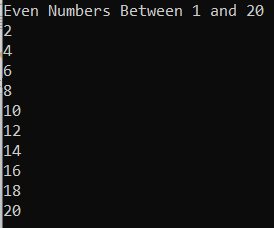Now, you can see the numbers are in the original order. Now, it doesn’t matter how many times you run the code, it will always bring up the current order of the elements, which is great in case you need that.

##### Difference Between OrderBy and AsOrdered Method in C#:

You might be interested in using the LINQ OrderBy method after the Where Extension method. But remember, the OrderBy method is used to sort the data, not to maintain the original order of the data. On the other hand, the AsOrdered method is used to maintain the original order of the elements. For a better understanding please have a look at the following example. The following example code is self-explained, so please go through the comment lines.

```using System;
using System.Collections.Generic;
using System.Linq;
namespace ParallelLINQDemo
{
class Program
{
static void Main(string[] args)
{
//Creating a Collection of integer numbers
List<int> numbers = new List<int>()
{
1, 2, 6, 7, 5, 4, 10, 12, 13, 20, 18, 9, 11, 15, 14, 3, 8, 16, 17, 19
};

//Using AsOrdered Method
//Fetching the List of Even Numbers using PLINQ
var evenNumbers1 = numbers
.AsParallel() //Parallel Processing
.AsOrdered() //Original Order of the numbers
.Where(x => x % 2 == 0)
.ToList();

Console.WriteLine("Even Numbers Between 1 and 20 using AsOrdered");
foreach (var number in evenNumbers1)
{
Console.WriteLine(number);
}

//Using OrderBy Method
//Fetching the List of Even Numbers using PLINQ
var evenNumbers2 = numbers
.AsParallel() //Parallel Processing
.Where(x => x % 2 == 0)
.OrderBy(x => x) //Sort the Elements in Ascending Order
.ToList();

Console.WriteLine("\nEven Numbers Between 1 and 20 using OrderBy");
foreach (var number in evenNumbers2)
{
Console.WriteLine(number);
}

}
}
}```
###### Output: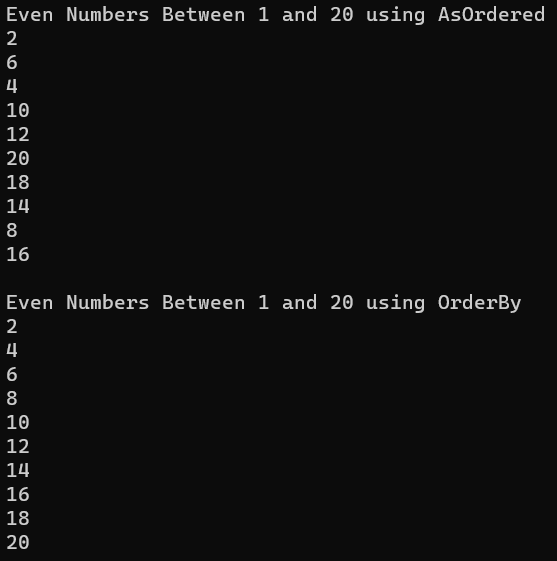As you can see in the above output, with the AsOrdered method, we are getting the data in its original order of the sequence. With the OrderBy method, we are getting the data in ascending order.

##### Maximum Degree of Parallelism and Cancellation Token in Parallel LINQ:

In Parallel Programming, we have discussed the maximum degree of parallelism and cancellation token. We can have the same functionality here with Parallel LINQ while processing a collection. For example, we can define the maximum degree of parallelism. We can also define and pass a cancellation token that will cancel the execution of the Parallel LINQ operation. For a better understanding, please have a look at the below image. Here, you can see, we are creating a CancellationTokenSource instance and setting the token to cancel the operation after 200 MilliSeconds. And then passing the Cancellation Token as a value to the WithCancellation method. Further, we are passing a value of 2 to the WithDegreeOfParallelism method which means a maximum of two threads going to process the operations.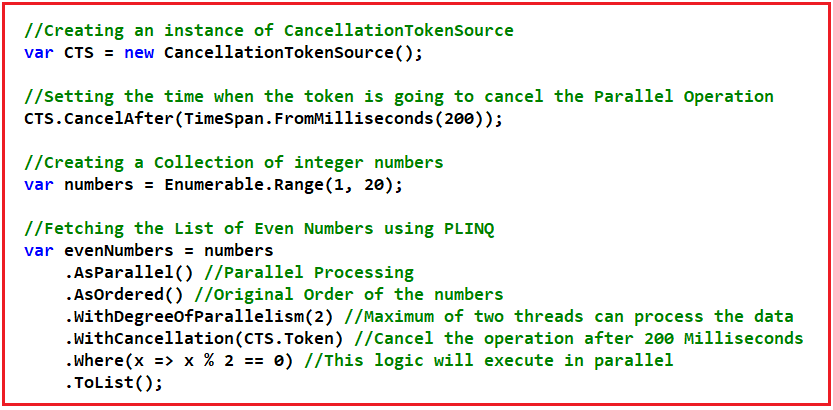So, with Parallel LINQ in C#, we can achieve the same functionality as parallel programming. The complete example code is given below. The following example code is self-explained, so please go through the comment lines for a better understanding.

```using System;
using System.Linq;

namespace ParallelLINQDemo
{
class Program
{
static void Main(string[] args)
{
//Creating an instance of CancellationTokenSource
var CTS = new CancellationTokenSource();

//Setting the time when the token is going to cancel the Parallel Operation
CTS.CancelAfter(TimeSpan.FromMilliseconds(200));

//Creating a Collection of integer numbers
var numbers = Enumerable.Range(1, 20);

//Fetching the List of Even Numbers using PLINQ
var evenNumbers = numbers
.AsParallel() //Parallel Processing
.AsOrdered() //Original Order of the numbers
.WithDegreeOfParallelism(2) //Maximum of two threads can process the data
.WithCancellation(CTS.Token) //Cancel the operation after 200 Milliseconds
.Where(x => x % 2 == 0) //This logic will execute in parallel
.ToList();

Console.WriteLine("Even Numbers Between 1 and 20");
foreach (var number in evenNumbers)
{
Console.WriteLine(number);
}

}
}
}
```
###### Output: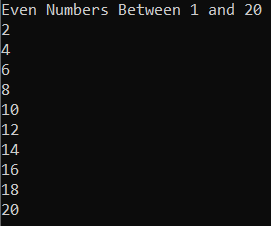##### Doing Aggregates in Parallel LINQ

Let us see how we can aggregate the elements of a sequence using Parallel LINQ in C#. For example, we can calculate the average of the elements of a collection, we can add the elements of a collection, etc. Let us see an example where we will calculate the Sum, Max, Min, and Average of a collection using Parallel LINQ in C#. The following example code is self-explained, so please go through the comment lines for a better understanding.

```using System;
using System.Linq;
namespace ParallelLINQDemo
{
class Program
{
static void Main()
{
var numbers = Enumerable.Range(1, 10000);

//Sum, Min, Max and Average LINQ extension methods
Console.WriteLine("Sum, Min, Max and Average with LINQ");

var Sum = numbers.AsParallel().Sum();
var Min = numbers.AsParallel().Min();
var Max = numbers.AsParallel().Max();
var Average = numbers.AsParallel().Average();
Console.WriteLine(\$"Sum:{Sum}\nMin: {Min}\nMax: {Max}\nAverage:{Average}");

}
}
}
```
###### Output: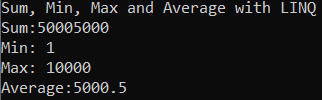##### Is Really Parallel LINQ Improving the Performance of an Application?

Let us see an example using both LINQ and Parallel LINQ to do the same task and then see the performance benchmark. Please have a look at the below example. In the below example, we are comparing the performance of LINQ and PLINQ Min, Max, and Average methods. The Min, Max, and Average methods are going to return a single scalar value or you can say aggregate value.

```using System;
using System.Diagnostics;
using System.Linq;

namespace ParallelLINQDemo
{
class Program
{
static void Main()
{
var random = new Random();
int[] values = Enumerable.Range(1, 99999999)
.Select(x => random.Next(1, 1000))
.ToArray();

//Min, Max and Average LINQ extension methods
Console.WriteLine("Min, Max and Average with LINQ");

Stopwatch stopwatch = new Stopwatch();
stopwatch.Start();
// var linqStart = DateTime.Now;
var linqMin = values.Min();
var linqMax = values.Max();
var linqAverage = values.Average();
stopwatch.Stop();

var linqTimeMS = stopwatch.ElapsedMilliseconds;

DisplayResults(linqMin, linqMax, linqAverage, linqTimeMS);

//Min, Max and Average PLINQ extension methods
Console.WriteLine("\nMin, Max and Average with PLINQ");
stopwatch.Restart();
var plinqMin = values.AsParallel().Min();
var plinqMax = values.AsParallel().Max();
var plinqAverage = values.AsParallel().Average();
stopwatch.Stop();
var plinqTimeMS = stopwatch.ElapsedMilliseconds;

DisplayResults(plinqMin, plinqMax, plinqAverage, plinqTimeMS);

}
static void DisplayResults(int min, int max, double average, double time)
{
Console.WriteLine(\$"Min: {min}\nMax: {max}\n" + \$"Average: {average:F}\nTotal time in milliseconds: {time}");
}
}
}
```
###### Output: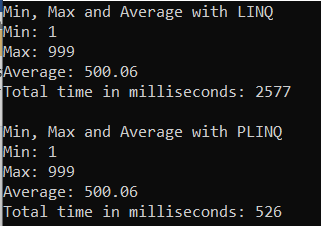##### Summary of Parallel Programming:
1. In this Parallel Programming Section, we saw that with parallelism, we can perform several actions at the same time on our computer. This helps with the speed of our program to solve certain problems.
2. We can use Task.WhenAll for IO-bound operations and a Parallel class for CPU-bound operations.
3. With Parallel For and Parallel Foreach, we can execute a loop in parallel where we cannot guarantee a defined order of executions.
4. We saw that it is not always convenient to use parallelism and this depends on the amount of work to be done. If it is very little work, the cost of parallelism is greater than not using it.
5. We can cancel operations in parallel and we can also define the number of threads to use by defining the maximum degree of parallelism.
6. We saw that atomic methods guarantee that there is no data corruption where multiple threads invoke the method concurrently.
7. A race condition is where multiple threads try to modify a variable at the same time causing unpredictable results.
8. Interlocked is a class that allows us to perform certain operations in an atomic way, such as adding variables that help us avoid race conditions.
9. The look allows us to create a block of code that can only be accessed by one thread at a time. In this way, we can avoid race conditions between multiple operations.
10. PLINQ allows us to use link syntax to process collections in parallel.

In the next article, I am going to discuss the Differences Between Multithreading vs. Asynchronous Programming vs. Parallel Programming in C# with Examples. Here, in this article, I try to Parallel LINQ (PLINQ) in C# with Examples. I hope you enjoy this Parallel LINQ (PLINQ) in C# with Examples.

### 2 thoughts on “Parallel LINQ in C#”

1.Guys,
Please give your valuable feedback. And also, give your suggestions about this Parallel LINQ in the C# concept. If you have any better examples, you can also put them in the comment section. If you have any key points related to Parallel LINQ in C#, you can also share the same.

1.Very Good Job,
I cleared all doubts about TPL.
Thank you so much